# Quarters of Shapes

Quarters of Shapes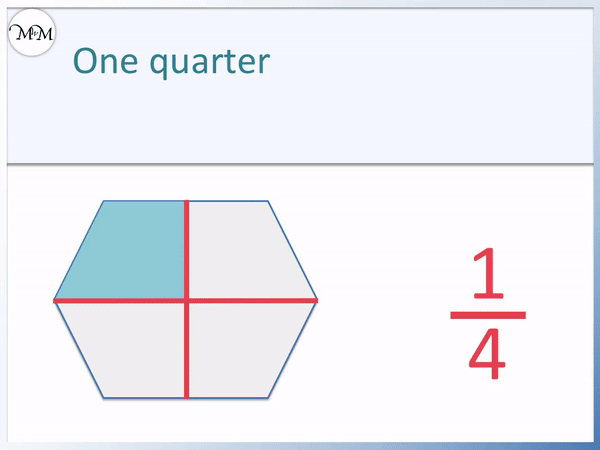• A quarter of a shape fits into the whole shape exactly four times.
• We can double our shaded area and double it again to make four copies.
• The shaded fraction fits into the whole shape exactly four times and so it is a quarter.
• A quarter means one out of four equally sized parts.
• Since all four of the divided sections are equally sized, this is a quarter.
• One quarter is written as a fraction by writing 1 out of 4 as   1 / 4  .

A quarter is one of four equally-sized parts.

A quarter of a shape fits into the whole shape exactly four times.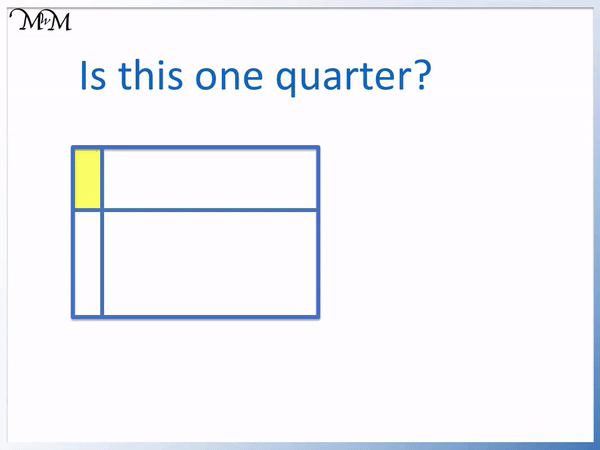• Is this shaded fraction equal to one quarter of the whole shape?
• This is a quarter if it fits into the whole shape exactly four times.
• We can double the region and double it again to make four of them.
• The fraction does not fill the whole shape when repeated four times and so it is not a quarter.
• This is less than one quarter because it is smaller than the other sections.
• If this was a quarter then all four regions would be equal in size.Supporting Lessons# Finding Quarters of Shapes

A quarter is one out of four equally sized parts.

A quarter is written as   1 / 4   as a fraction and 0.25 as a decimal.

If a fraction of a shape fits into the whole shape exactly four times then it is a quarter.

The shape below is a square. To find a quarter of this square we will divide it into four equally sized parts.The square shape is divided into four equally sized sections using the red cross shown, halving the square and halving it again.

We shade in one of these four sections to show that it is a quarter.

We have ‘one out of four’ equally sized parts and this can be written as a fraction as 1 / 4.

This fraction can be read as ‘one out of four’ and it also means 1 ÷ 4, which means that the one whole shape has been divided into 4.

In the example below we will find one quarter of a circle shape.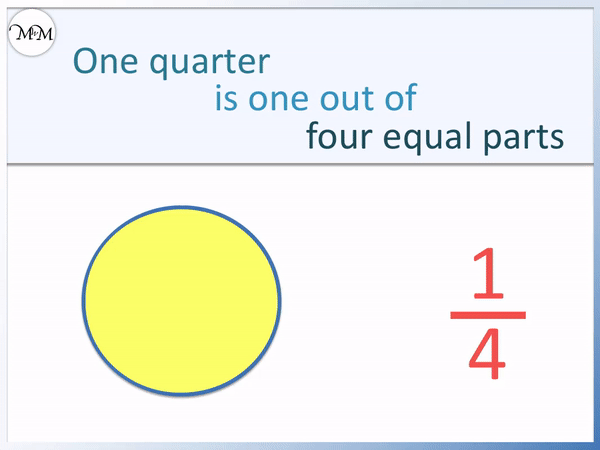One quarter is one out of four equally sized parts and so we can see a quarter visualised above.

Again the red cross shown has been useful in finding this quarter as we were able to halve the shape and halve it again.

When teaching finding one quarter to children (a common year 1 primary school task), we usually start with easier shapes. We can first look to see if the shape can be halved and halved again by dividing it directly through the middle horizontally and vertically.

This will often be the case for many year 1 questions.

Again this hexagon shape can be quartered in this way, dividing it through the middle horizontally and vertically.We have one out of four equally sized parts. The shaded region fits into the whole shape exactly four times and we can see that all of the four regions are equal in size.When teaching quarters of shapes, it can be useful to show the shaded quarter fitting in exactly four times to fill the whole shape. You can fold the shape, or use tracing paper to make copies of the quarter to duplicate.

We can see this in the example of the circle below.

You can also think of this as doubling the shaded region and doubling it again. Doubling a quarter makes a half and then doubling a half makes a whole.The same technique is shown for the quarter of the square shape below: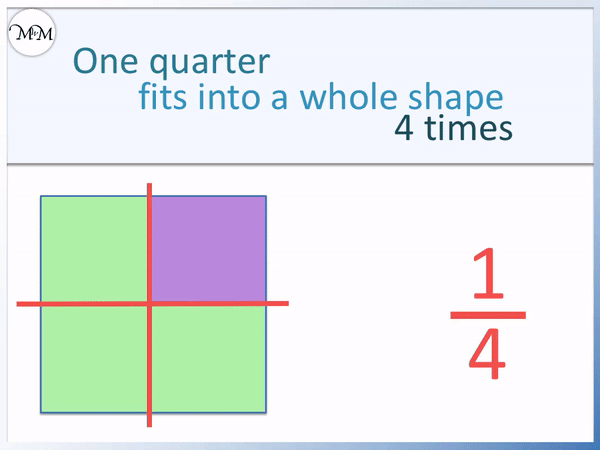These shaded regions seen so far all fit exactly into the whole shape four times.

Sometimes we may be asked if a region is equal to a quarter or not.

A common mistake for children first introduced to quarters is that they may simply count 4 separate regions, without checking if the 4 regions are equal in size.

To be a quarter, the four regions must be equal in size.

Is the following region the same as the fraction of   1 / 4   ?We can fit 4 of the shaded regions together and we see that there is still space within the whole shape.

Four of the shaded regions do not fill the whole shape exactly and so this is not one quarter.

Since less than the whole shape is filled, the shaded region is less than one quarter.

Comparing the sizes of the regions, the shaded region is smaller than some of the other regions and so this is another way to see that this is less than one quarter.

We do not have 4 equally sized regions.

In the example below we are asked to decide if we have one quarter of the whole shape.We do not have 4 equally sized sections and so, this is not a quarter.

We can place 4 of the parts together and they fill more than the whole shape. Therefore this is more than one quarter.

We can see that although we have four sections, the shaded section is larger than some of the others.Now try our lesson on Shading Fractions of Shapes where we learn how to shade any given fraction of a shape.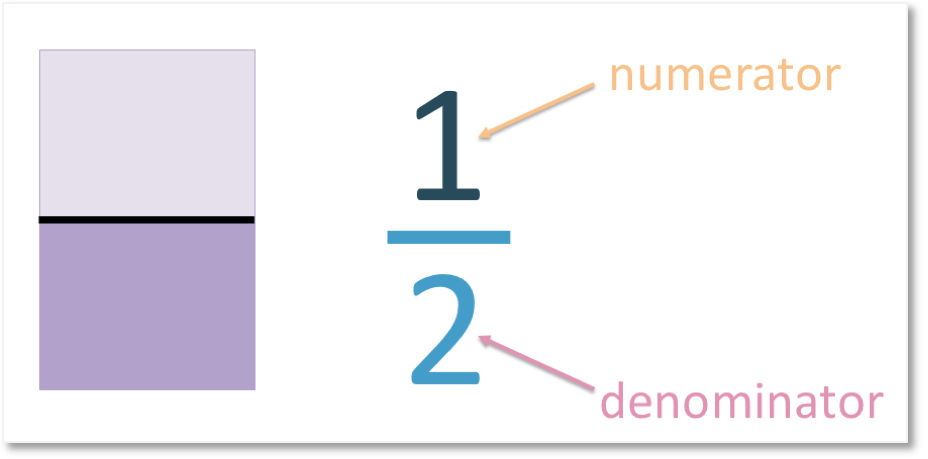error: Content is protected !!